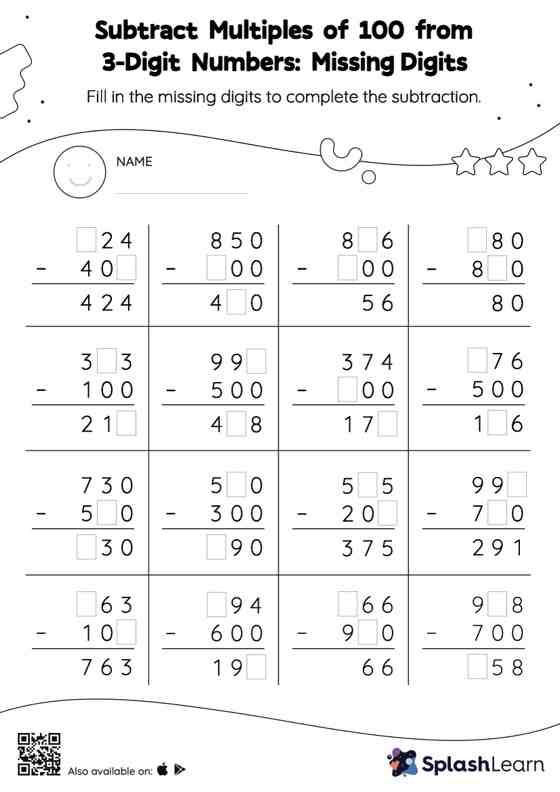# Subtract Multiples of 100 from 3-Digit Numbers: Missing Digits Worksheet

Home > Subtract Multiples of 100 from 3-Digit Numbers: Missing DigitsThis worksheet consists of a set of questions on subtraction where students are required to subtract multiples of 100 from 3-digit numbers. In this worksheet, students practice solving problems using the column method. This method is especially helpful with problems involving bigger multi-digit numbers as the format provides an easy structure to follow.